`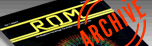ROM MAGAZINE ISSUE 4 — FEBRUARY/MARCH 1984 / PAGE 31`

 POLAR GRAPH PLOTTER By TUONG (Tom) TRAN     To plot a polar graph with a pencil and paper takes a lot of time, but with a computer this is an easy task. By using graphics mode 8 on the ATARI it s quite simple to make some excellent looking polar graphs. This program is a very helpful teaching tool because a polar graph can be plotted very quickly by just entering in your function.     Suppose we had a relation between R, the distance from a point called pole, and T(Greek symbol theta), the angle measured counter clockwise from the polar axis. The pole is analogous to the origin axis and lies along the X-axis. The relation between R and T(heta) is usually described by an equation of the form: R=F(T) The equation: R=90*SIN(2*T) is one example. Refer to Diagram 1 for an illustration of some of these concepts, including a graph of the equation R=90*SIN(2*T), called a four-leaved rose.     The key to using a computer to graph polar coordinates is the transformation formulas: X=R*COS(T) Y=R*SIN(T) and of course, the computer's ability to perform a PLOT X,Y instruction.     Below are eight other diagrams with their functions that you can type into the program. DIAGRAM 1. A Four-Leaved Rose. R=90*SIN(2*T)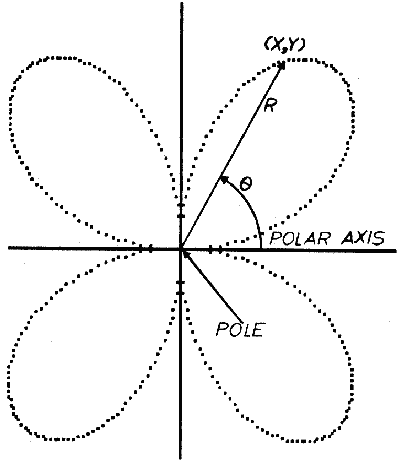DIAGRAM 2. A Three-leaved Rose. R=80*SIN(3*T)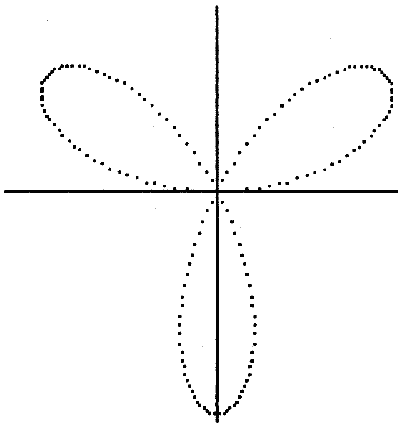DIAGRAM 3. A Thirteen-Leaved Rose. R=80*SIN(3*T)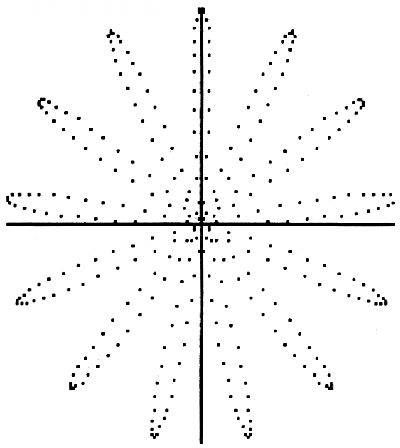DIAGRAM 4. Bear-Head. R=25*(2+SIN(3*T))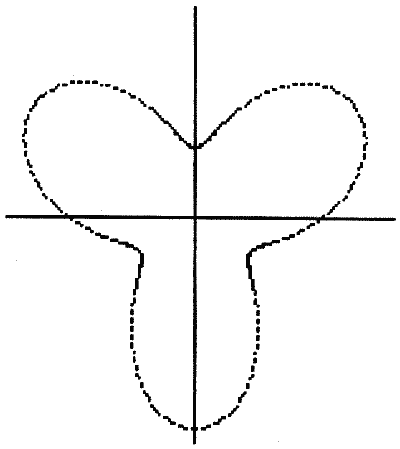DIAGRAM 5. The Cardioid. R=40*(1+COS(T))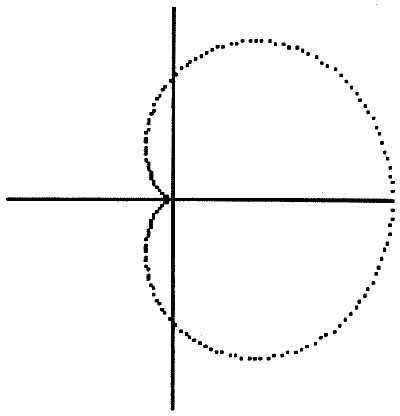DIAGRAM 6. The Spiral of Archimedes. R=0.05*T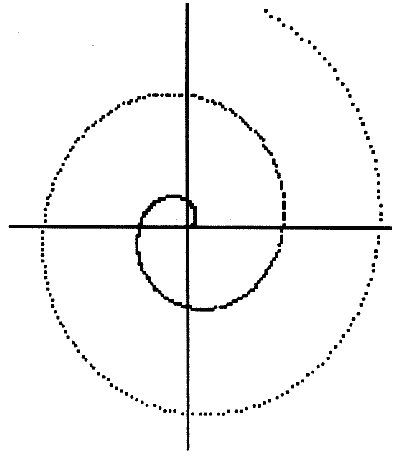DIAGRAM 7. Inward Circle. R=80*COS(T/3)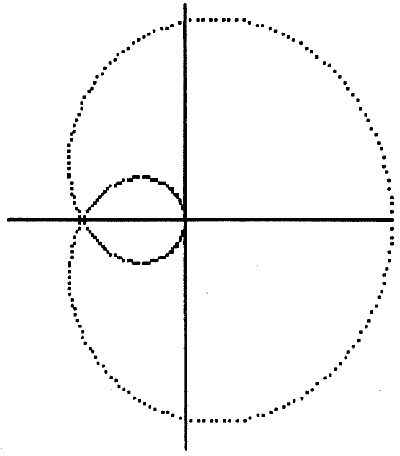DIAGRAM 8. Swirl. R=SQR(360000/T)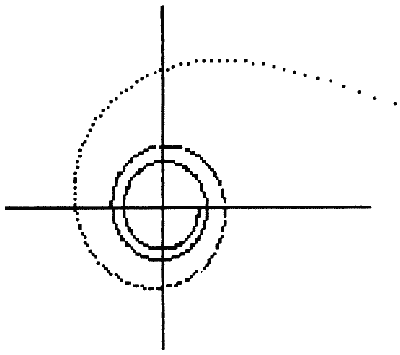The program below allows one to type in a polar graph function and watch the graph draw on the screen. It uses EQ\$ to store the function you enter so that if you wish to go back to it to edit it you can. A sample function is given at line 0 in order to show you the form. When you enter in a function that the computer doesn't understand it will give you an error message that will flash on the screen. You then can go back and edit the function you typed in. After you enter in your function the program will ask you if you want to set the increment. If you say no it will set the increment at 1. If you say yes you can tell it how large of an increment and how many revolutions that you want it to go. Remember for Theta all you need to type in is a T so that there could be room for larger functions. If you get any interesting graphs feel free to send in the functions. 1 REM ******************************* 2 REM * TWO DIMENSIONAL POLAR PLOTS 3 REM * WRITTEN BY: Tom Tuong Tran 6 REM ******************************* 9 DEG :REM -THIS PROGRAM WILL WORK IN DEGREE 10 ? "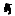":DIM FUC\$(60),EQ\$(60),A\$(3) 20 ST=PEEK(560)+256*PEEK(561)+5 30 FOR I=1 TO 2:POKE ST+I,6:NEXT I 40 FOR I=3 TO 4:POKE ST+I,7:NEXT I 50 FOR I=5 TO 11:POKE ST+I,6:NEXT I 60 FOR I=11 TO 17:POKE ST+I,2:NEXT I 70 FOR I=18 TO 20:POKE ST+I,6:NEXT I 80 POKE ST+20,PEEK(560) 90 POKE ST+21,PEEK(561) 100 ? "****************";? "TWO DIMENSIONAL POLAR PLOTS":? : ? "****************" 110 POKE 85,1:? "BY: TOM TUONG TRAN" 120 ? "This program will plot the graph of any given function having the form:":POKE 85,33:? "R=F(T)" 130 ? "EXAMPLE: R=80*COS(6*T) or R=80*SIN(T/3)" 140 ? "for R=Radius and T=Angle in Degree(s)" 150 POKE 85,0:? "PRESS OPTION TO EXIT OR START TO BEGIN" 160 IF PEEK(53279)=3 THEN GRAPHICS 0:END 170 IF PEEK(53279)=6 THEN ? "":GOTO 190 180 GOTO 160 190 EQ\$="80*COS(3*T)" 200 POKE 710,96:COLOR 5 210 GRAPHICS 0:? "YOU CAN PLOT THE TRIGONOMETRIC FUNCTION BY ENTERING EACH FUNCTION IN THE FORM :" 220 ? "             R=F(T)" 230 ? "     FOR     R=RADIUS          and T =ANGLE IN DEGREE(S)" 231 ? "↓↓*********************************":TRAP 200 232 ? "↓↓  1) EDIT PREVIOUS FUNCTION.":? "↓    2) RUN PREVIOUS FUNCTION.":? "    3) ENTER NEW FUNCTION." ↓ 240 ? "↓    4) QUIT.":? "↓↓WHICH ";:INPUT OPTION 250 ON OPTION GOTO 280,400,280,270 260 GOTO 200 270 GRAPHICS 0:? "":GOTO 20 275 REM --ENTER-FUNCTION------ 280 OPEN #1,4,0,"E:" 290 POKE 710,100:POKE 712,100:POKE 709,110 300 ? "↓↓    TYPE IN THE FUNCTION R=F(T)":? "R="; 305 IF OPTION=1 THEN ? EQ\$:POKE 82,4:? "" 310 INPUT #1,EQ\$:? "":POKE 82,2 320 CLOSE #1:FUC\$="550 R=" 330 POSITION 2,5:POKE 709,148 340 FUC\$(LEN(FUC\$)+1)=EQ\$ 350 ? FUC\$:POSITION 2,10 360 ? "CONT" 370 POSITION 0,2:POKE 842,13:STOP 380 POKE 842,12:POKE 709,202:? "£":POKE 710,100:POKE 712,100:POKE 709,110 400 ?↓SET INCREMENT & REVOLUTION (Y/N)" 410 POKE 764,255:TRAP 670 420 K=PEEK(764):IF K=43 THEN POKE 764,255:GOTO 450 430 IF K=35 THEN POKE 764,255:I=1:RE=1:GOTO 470 440 GOTO 420 450 ? "↓ INPUT INCREMENT ";:INPUT I 460 ? " INPUT NUMBER OF REVOLUTION ";:INPUT RE 465 REM -SET UP SCREEN AND DRAW AXIS 470 GRAPHICS 8:T=0:C=0 480 POKE 710,96:COLOR 5 490 PLOT 159,0:DRAWTO 159,159 500 PLOT 0,79:DRAWTO 319,79:TAB=(40-(LEN(EQ\$)+11))/2:IF TAB <0 THEN TAB=0 510 POKE 752,1:POKE 82,TAB:? :? "FUNCTION R=":EQ\$:POKE 82,2:IF TAB>O THEN ? :? "    PRESS TO EXIT" 515 REM 520 REM -PLOT THE GRAPH------- 525 REM 530 T=T+I 540 IF T>=C*360 THEN C=C+1:T=T+I/RE:? "REV=";C;"" 550 R=80*COS(3*T) 560 X=R*COS(T) 570 Y=R*SIN(T):? "X=";INT(X);"  ":? "Y=";INT(Y);"  " 580 IF X+159>319 OR X+159<0 OR 79-Y>159 OR 79-YRE*360 THEN 630 610 IF PEEK(764)<>255 THEN POKE 764,255:GOTO 630 620 GOTO 530 630 ? CHR\$(253);"↓ WOULD YOU LIKE TO TRY THIS FUNCTION AGAIN R=";EQ\$;" (Y/N)":POKE 764,255 640 IF PEEK(764)=43 THEN POKE 764,255:? "":POKE 752,0:GOTO 400 650 IF PEEK(764)=35 THEN POKE 764,255:POKE 752,0:GOTO 200 660 GOTO 640 665 REM -ERROR MESSAGE-------- 670 ? "↓    ERROR-  In the FUNCTION":? "↓     PLEASE TRY AGAIN" 680 FOR Q=l TO 30:? "↑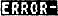":FOR W=1 TO 10:NEXT W:? "↑   ERROR-":FOR W=1 TO 10:NEXT W:NEXT Q:GOTO 190